### Refractive index profile

 OptiLayer displays refractive index profile in various forms: Analysis --> Refractive index profile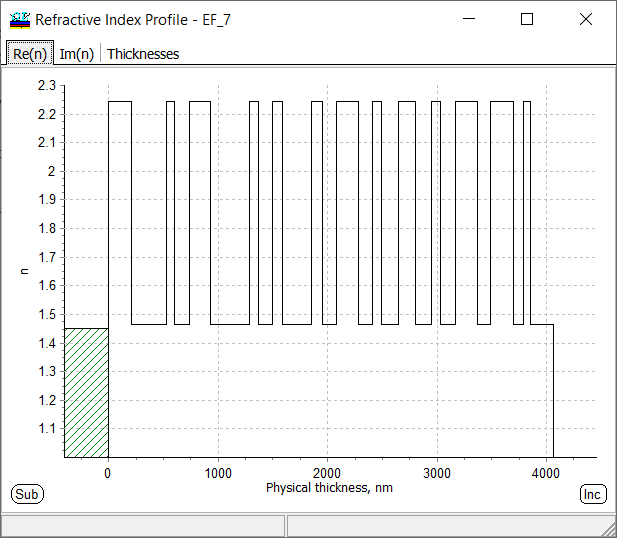Fig. 1. Refractive index profiles of a 26-layer design: $$n(z)$$ form. Refractive index of an optical coating can be represented as a function $$n(z)$$ (Fig. 1). Refractive index values are shown at the reference wavelength.  Important: In OptiLayer, layers are numbered starting from the substrate side. Typically, $$n(z)$$ is a step function taking high- and low-index values, $$n_H$$ and $$n_L$$. At the same time, $$n(z)$$ can be an arbitrary function as, for example, in the case of rugate filters. Alternative form of refractive index profile representation is bar diagram form, where thicknesses of layers of each layer material are represented as bars of different color. Refractive index profile is re-plotted in the course of design process.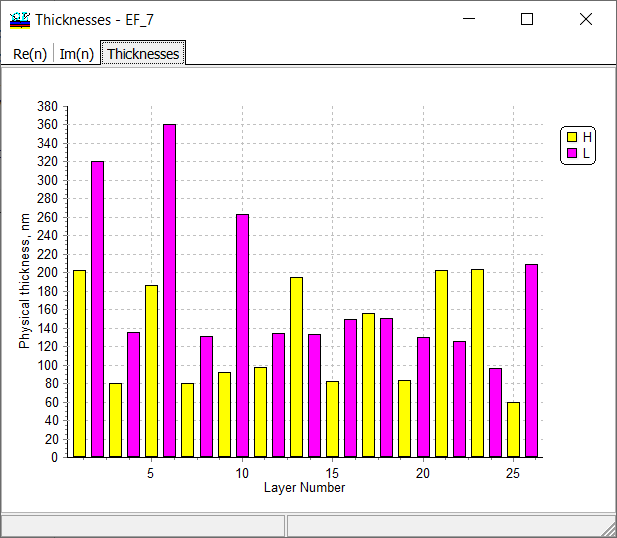Fig. 2. Physical thicknesses of a 26-layer design.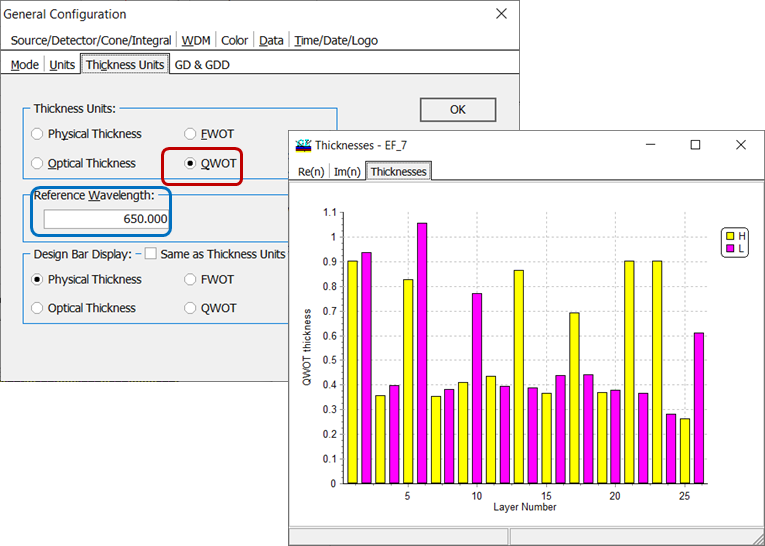Fig. 3. Switching refractive index profile into QWOT representation.Specification of the reference wavelength. Refractive index profiles can be represented in the form of optical thicknesses, quarter-wave (QWOT, Fig. 3) and full-wave (FWOT) optical thicknesses. Optical thickness $$nd$$ of a non-absorbing layer is calculated as: $nd=n(\lambda_0)\cdot d,$ where $$\lambda_0$$ is the reference wavelength specified in General Configuration menu (Fig. 3). QWOT means that optical thickness of a layer is equal to $$\lambda/4$$: $nd=\lambda_0/4.$ In Thickness Units tab, you can switch between different representations of layer thicknesses. OptiLayer allows you to plot a refractive index profile for the design currently loaded into the memory. Using Chart Editor, you can change the colors, line widths, axis etc. Also, you can easily export profiles in numerical or graphical form into files of various formats (xls, png, jpeg, etc).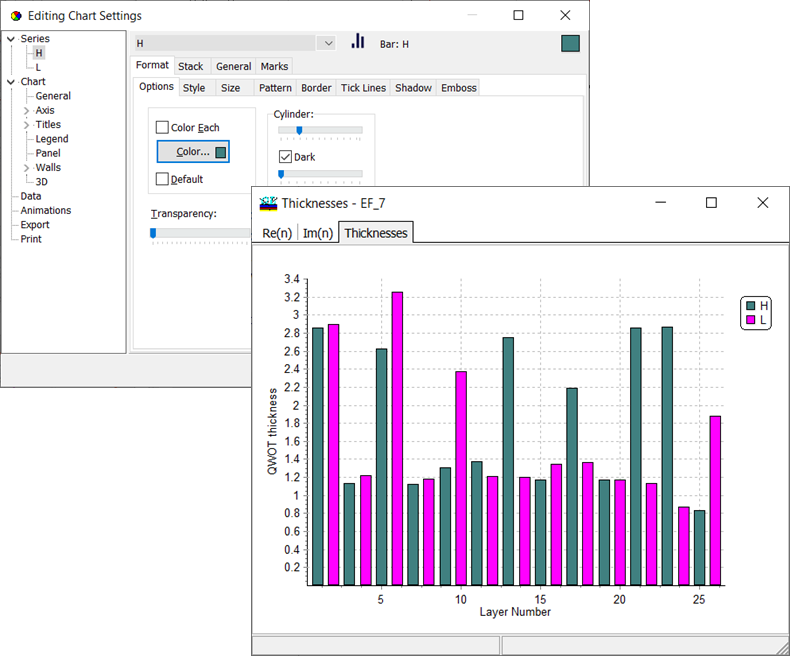Fig. 4. For your convenience, you can change colors, line width etc. on all diagrams.

### Easy to startOptiLayer provides user-friendly interface and a variety of examples allowing even a beginner to effectively start to design and characterize optical coatings.        Read more...

### Docs / SupportComprehensive manual in PDF format and e-mail support help you at each step of your work with OptiLayer.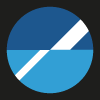If you are already an experienced user, OptiLayer gives your almost unlimited opportunities in solving all problems arising in design-production chain. Visit our publications page and challenge page.